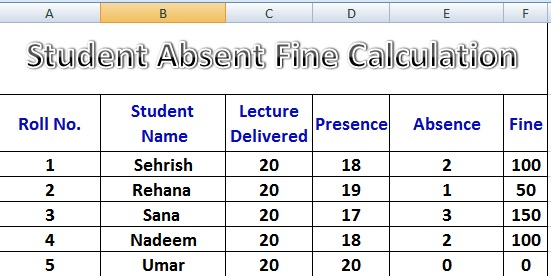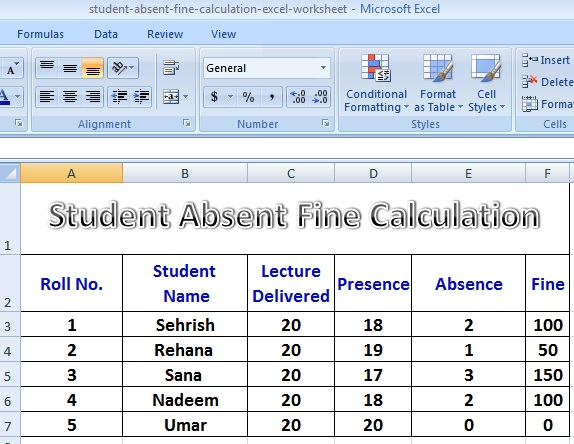# Perfect Computer Notes

Super Easy Short Computer Notes To Improve Computer Literacy and Your IT Skills

# Excel Worksheet Student Absence Fine Calculation Formulas

Today, we will discuss how to create an Excel Worksheet that helps calculate Students Absence Fine Calculation on basis of Total Lectures Delivered and Total Lectures Attended (Presence) of the student.Excel Worksheet Student Absence Fine Calculation Formulas

### Steps To Prepare an Excel Worksheet for Student Absence Fine Calculation

1. Start MS Excel with a blank workbook option.
2. Type sample data in first Excel worksheet as shown in the given image.
3. Type formula for calculation of number of absence of a student.
Place cursor in Cell with reference E3.
Type C3-D3 and press Enter### Steps To Prepare an Excel Worksheet for Student Absence Fine Calculation

4. Copy the formula of cell E3 in all lower level cells with the help of Fill Handle in
lower right corner of Cell E3.
5. Type formula for calculation of fine by multiplying cell E3 with 50.
6. Type formula of fine calculation =E3 * 50 and press enter in cell referenced by F3.
7. Copy the fine calculation formula downward in all required cells of column F.
8. Congratulations you have successfully prepared Excel Worksheet for Absence Fine calculation of         students.
9. Please, do not forget to save file.